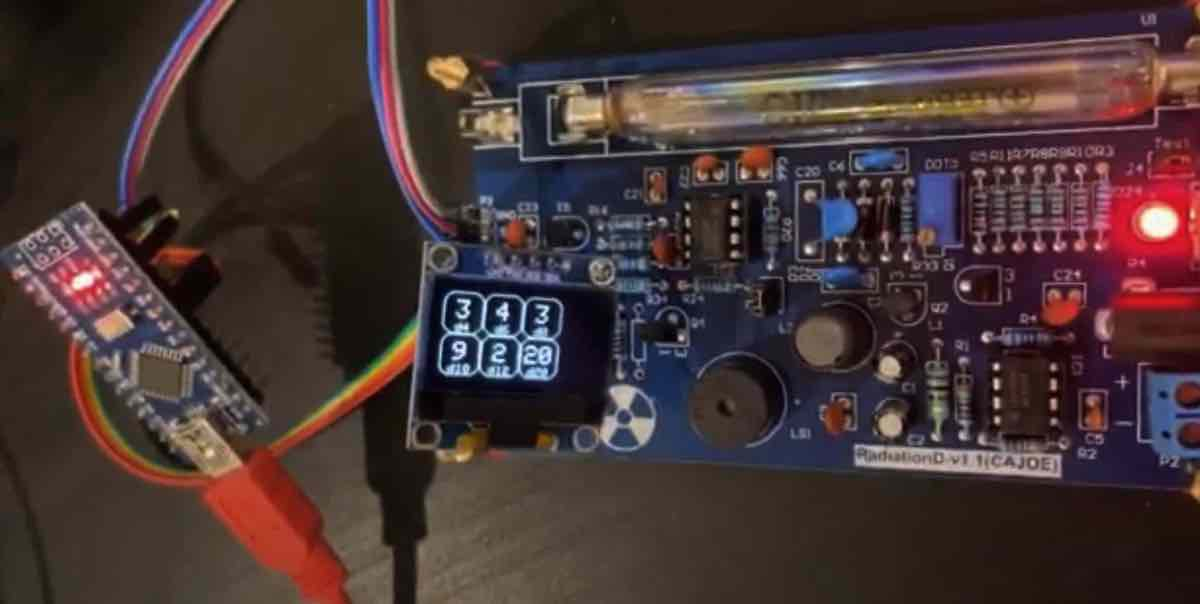# Gerry's World

A glimpse into my lifeJuly, 2022

# Current progress:

• ✅ turn on / test Geiger kit
• ✅ prototype with jumper cables
• ✅ test connection w/ Arduino Nano
• ✅ implement math/code to efficiently convert geiger detections to random dice rolls
• ✅ display results on OLED
• ⬜️ create button inputs
• ⬜️ create PCB
• ⬜️ schematic (~75% done)
• ⬜️ create board layout

# Unfinished documentation on random number sampling math

### (These are from text messages)

Sorry I half replied to this and forgot to finish

The probability of a detection occurring at any equally sized time window is the same, so say for example, you split 6ms into 6 pieces (1 for each die face). If a detection occurs in the first 1ms, it’s a 1. If in the second, it’s a 2, etc. But what if a detection doesn’t occur in any of them, or if a detection occurs in multiple of them? The easiest thing to do is to just throw out the whole 6ms of data and start over again. Note: This method is DIFFERENT that just taking the time between two successive detections and taking mod 6. This is saying the start time is fixed, and you can’t stop at the first detection, you have to let it run until the end of the 6ms cycle and if you are unlucky and get another detection, then you have to throw out that data. This works fine, but since there’s only 1 detection per 2.3 seconds on average for the geiger tube I have, I want to be a bit more efficient with my detections. So I do 2 things:

First, most importantly, I save the timings of previous detections in non-volatile memory so that there’s always like 250 old random numbers queued up ready to go.

Second, I recycle some cases. For example, if there were 2 different detections within the 6ms interval, then there’s (6 choose 2) = 6!/2!/4! = 15 different ways that’s possible, so I assign a canonical numbering to number all possible pairs of detections. If the number is 1 or 7, then that’s a dice roll of 1. If it’s 2 or 8, then that’s a 2. And so on. Anything 13 or above means I have to throw out this 6ms of data, but now instead of throwing out every single case of 2 detections, I only throw out 3/15 = 20% of the cases, so it’s more sample efficient. And I do a similar procedure for 3, 4, 5 detections. (0 detections and 6 detections there’s only 1 possible way so that’s just discarded anyway). This isn’t that big of a deal for a single 6-sided die, but actually with a single detection I generate 4, 6, 8, 10, 12, 20 sided dice all simultaneously which is the equivalent of a 460,800 sided die. Because I only having timing resolution of 4 microseconds, I calculated that on average if I don’t use the transformation then the expected value for the amount of time required for a new dice roll is ~5.5s, whereas with the transformation it’s only 2.3s. And it turns out that, with the transformation, pretty much any number of sides of dice actually take the same expected amount of time up to about 1 million-sided die

In actuality just throwing out any time where there’s not exactly 1 detection per trial would be practically more than good enough since I queue up a bunch of old data in non-volatile memory, but doing that math is just kind of fun

oh also with a 460,800 sided die, then if there are e.g. 3 detections within the same trial, then you actually get more than one dice roll at a time, since there’s so many options that it’s greater than 460800^2 so you get 3 rolls at a time

I wrote a program to try to find the optimal number of ”buckets”s” since it might be different than the actual number of sides on the dice that you care about, but since the probability of getting a detection within any 4us time slice is so minuscule, then it turns out to just always be best to use the same number of “buckets” as dice sides

So let me clarify the algorithm:

Define N the number of dice faces, and T the time resolution of your measuring device (for me 4us)

MAIN:

1. Start the clock
2. Wait for N*T seconds, recording the times t1, t2, … that a detection occurs.
3. Convert the times t1, t2, … into dice rolls n1, n2, … using CONVERT
4. Repeat

CONVERT: Denote d is the number of detections t1, t2, …, td

1. Compute NUM equals the total number of ways to achieve d detections: (N choose d) = N! / d! / (N-d)!
2. Compute key is the index of {t1, t2, …, td} using the canonical ordering. (Compute as the total number of ways to achieve d detections such that the i’th detection is smaller than ti)
3. While NUM >= N:
4. Compute THRESH is the largest multiple of N <= NUM
5. If key > THRESH, exit this function without returning any more data
6. yield a dice roll: key mod THRESH
7. Assign NUM = NUM / N
8. endwhile